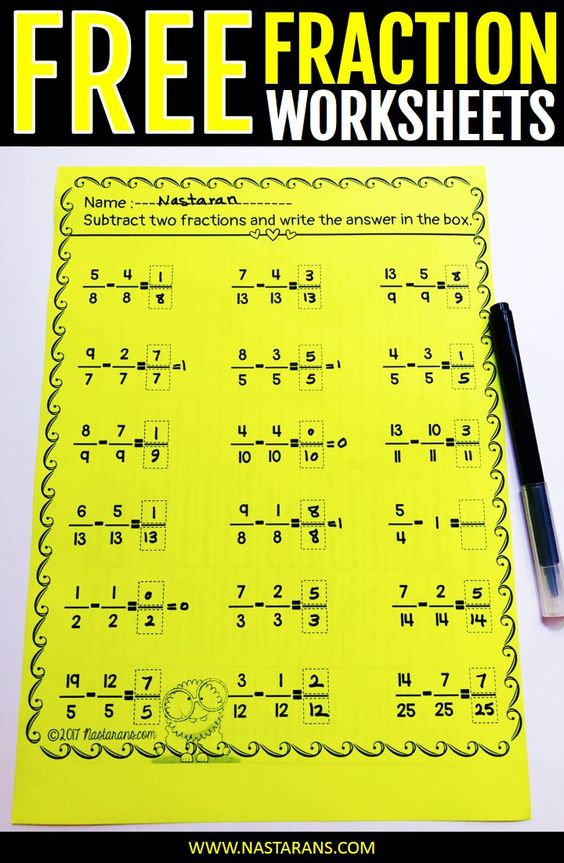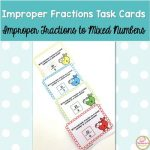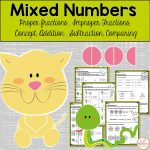# Mixed Numbers and Improper Fractions Worksheets FreeFree mixed Numbers and Improper Fractions Worksheets contain four pages and these are perfect for the students in fifth grade, and you can use this for reinforcing your students in 6th grade.

• Comparing Fractions
• Fractions Subtraction
• Improper fractions to mixed numbers

### You may also like:40 task cards help you teach conversion between mixed numbers and improper fractions. Click Here to check out Improper Fractions To Mixed Numbers Task Cards.Each card has a mixed number, and the students convert this mixed number into the improper fraction and then save their answers in the answer sheets. Click Here to check out Mixed Numbers To Improper Fractions Task Cards.Fractions For Third Grade includes 21 printable worksheets that cover Identifying fractions,Fractions in a set, Equal parts, Fractions on the number-line, Equivalent fractions, Cut and paste fractions,Comparing fractions Click to Here check out 3rd Grade Fractions Worksheets.Contains 27 worksheets that covers proper fraction review, improper fraction, comparing fractions,mixed number concept, adding and subtracting fractions, converting improper fractions to mixed number, and converting mixed numbers to improper fractions. Answer key also included! Click Here to check out Mixed Numbers and Improper Fractions.29 printable worksheets that cover Fraction Concept, Equal/non equal parts, Fractions on the number-line, Equivalent fractions,Comparing fractions, Answer Key Click Here to check out Fractions Worksheets :No preparation.Free Mixed Numbers And Improper Fractions Worksheets Click Here to check out

If you like this resource, you may find it HERE or the picture below.

### Pin for later:## Similar Posts

This site uses Akismet to reduce spam. Learn how your comment data is processed.